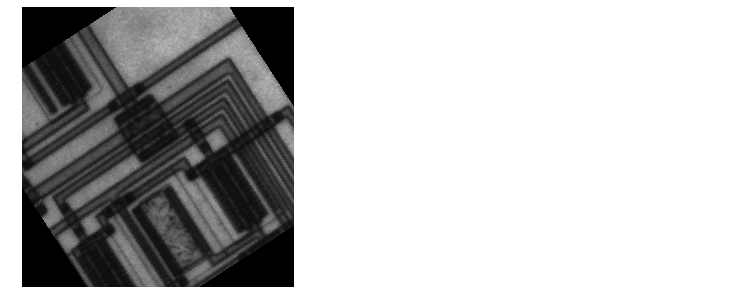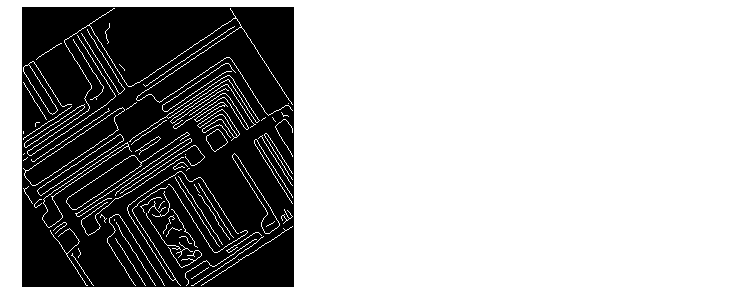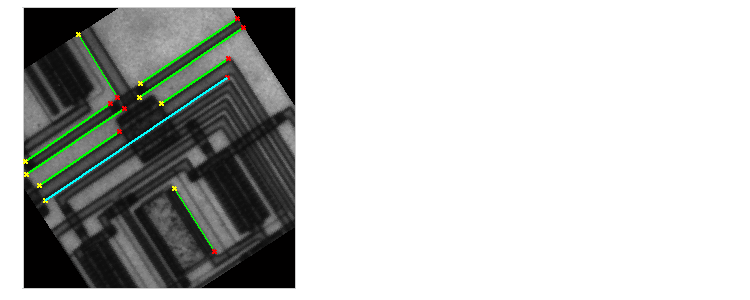Image Processing Toolbox User's GuideDetecting Lines Using the Hough Transform

The Image Processing Toolbox includes functions that support the Hough transform.

• hough
• houghpeaks
• houghlines

The hough function implements the Standard Hough Transform (SHT). The Hough transform is designed to detect lines, using the parametric representation of a line:

• rho = x*cos(theta) + y*sin(theta)


The variable rho is the distance from the origin to the line along a vector perpendicular to the line. theta is the angle between the x-axis and this vector. The hough function generates a parameter space matrix whose rows and columns correspond to these rho and theta values, respectively.

The houghpeaks function finds peak values in this space, which represent potential lines in the input image.

The houghlines function finds the endpoints of the line segments corresponding to peaks in the Hough transform and it automatically fills in small gaps.

The following example shows how to use these functions to detect lines in an image.

1. Read an image into the MATLAB workspace.
• I  = imread('circuit.tif');

2. For this example, rotate and crop the image.
• rotI = imrotate(I,33,'crop');3. Find the edges in the image.
• BW = edge(rotI,'canny');4. Compute the Hough transform of the image using the hough function.
• [H,theta,rho] = hough(BW);

5. Display the transform.
• imshow(H,[],'XData',theta,'YData',rho,...
'InitialMagnification','fit');
xlabel('\theta'), ylabel('\rho');
axis on, axis normal, hold on;6. Find the peaks in the Hough transform matrix, H, using the houghpeaks function.
• P = houghpeaks(H,5,'threshold',ceil(0.3*max(H(:))));

7. Plot the peaks.
• x = theta(P(:,2));
y = rho(P(:,1));
plot(x,y,'s','color','white');

8. Find lines in the image.
• lines = houghlines(BW,theta,rho,P,'FillGap',5,'MinLength',7);

9. Create a plot that superimposes the lines on the original image.
• figure, imshow(rotI), hold on
max_len = 0;
for k = 1:length(lines)
xy = [lines(k).point1; lines(k).point2];
plot(xy(:,1),xy(:,2),'LineWidth',2,'Color','green');

% Plot beginnings and ends of lines
plot(xy(1,1),xy(1,2),'x','LineWidth',2,'Color','yellow');
plot(xy(2,1),xy(2,2),'x','LineWidth',2,'Color','red');

% Determine the endpoints of the longest line segment
len = norm(lines(k).point1 - lines(k).point2);
if ( len > max_len)
max_len = len;
xy_long = xy;
end
end

% highlight the longest line segment
plot(xy_long(:,1),xy_long(:,2),'LineWidth',2,'Color','cyan');Tracing Boundaries Using Quadtree Decomposition